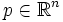# Sphere in Euclidean space

Consider Euclidean space$\R^n$. Let$p \in \R^n$ be a point and$r$ be a nonnegative real number. The sphere with center$p$ and radius$r$ in$\R^n$ is defined as the set of those points in$\R^n$ that are at distance exactly$r$ from$p$.
• A sphere of radius zero is just a point. Any other sphere is a$(n-1)$-dimensional manifold.
• Given two spheres of the same radius, the translation map from$\R^n$ to$\R^n$ that takes the first center to the second center, also takes the first sphere to the second sphere. In other words, given two spheres of the same radius, there is an isometry of$\R^n$ taking one to the other.
• The orthogonal group$O_n(\R)$ maps every sphere centered at the origin, to itself.